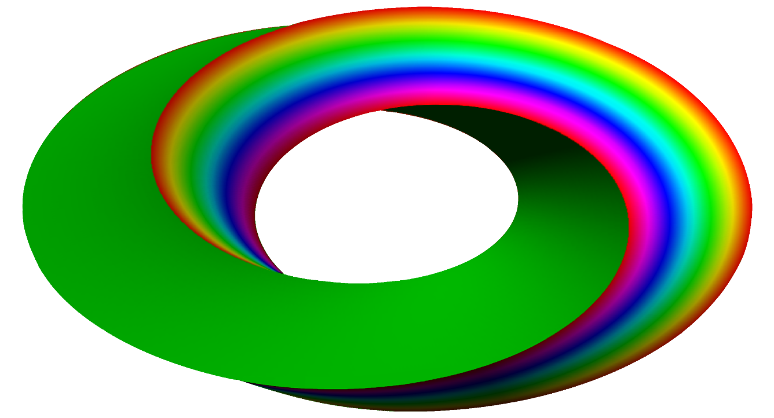# Projects

### A: Stochastic and dynamical processes

 A1 Spectral theory of aperiodic order A2 Numerical analysis of high dimensional transfer operators A3 Stochastic dynamics and bifurcations A4 Asymptotics of spectral distributions A5 Stochastic evolutions in continuum A6 Analysis and stochastic processes on metric measure spaces A8 Fine properties of long-range operators and processes A9 Dynamics and asymptotic behaviour of stochastic evolution systems A10 Nonlocal operators

### B: Spectral structures

 B1 Asymptotic distributions, lattices and groups B2 Combinatorial and topological structure of aperiodic tilings B3 Numerical analysis of equivariant evolution equations B4 Kolmogorov operators and SPDE B5 Algebraic geometry, cohomology and abelian varieties B6 Invariant harmonic analysis and Selberg zeta functions B7 Analysis of discretization methods for nonlinear evolution equations B8 Initial value problems for nonlinear dispersive equations at critical regularity

### C: Topological methods in algebra

 C1 Gauge theoretical methods in manifold theory C2 Linear algebraic groups over arbitrary fields C3 Topological and spectral structures in representation theory C4 Milnor conjecture, Galois cohomology and algebraic cobordism C5 p-adic symmetric spaces, p-adic uniformisation and L-functions C6 Groups, buildings, and model theory C7 Automorphic representations and their local factors C8 Finiteness Properties of Infinite Discrete Groups C10 Local cohomology and support in representation theory C11 Algebraic and analytic aspects of holomorphic Lagrangian fibrations C12 Representation growth of arithmetic groups C13 The geometry and combinatorics of groups